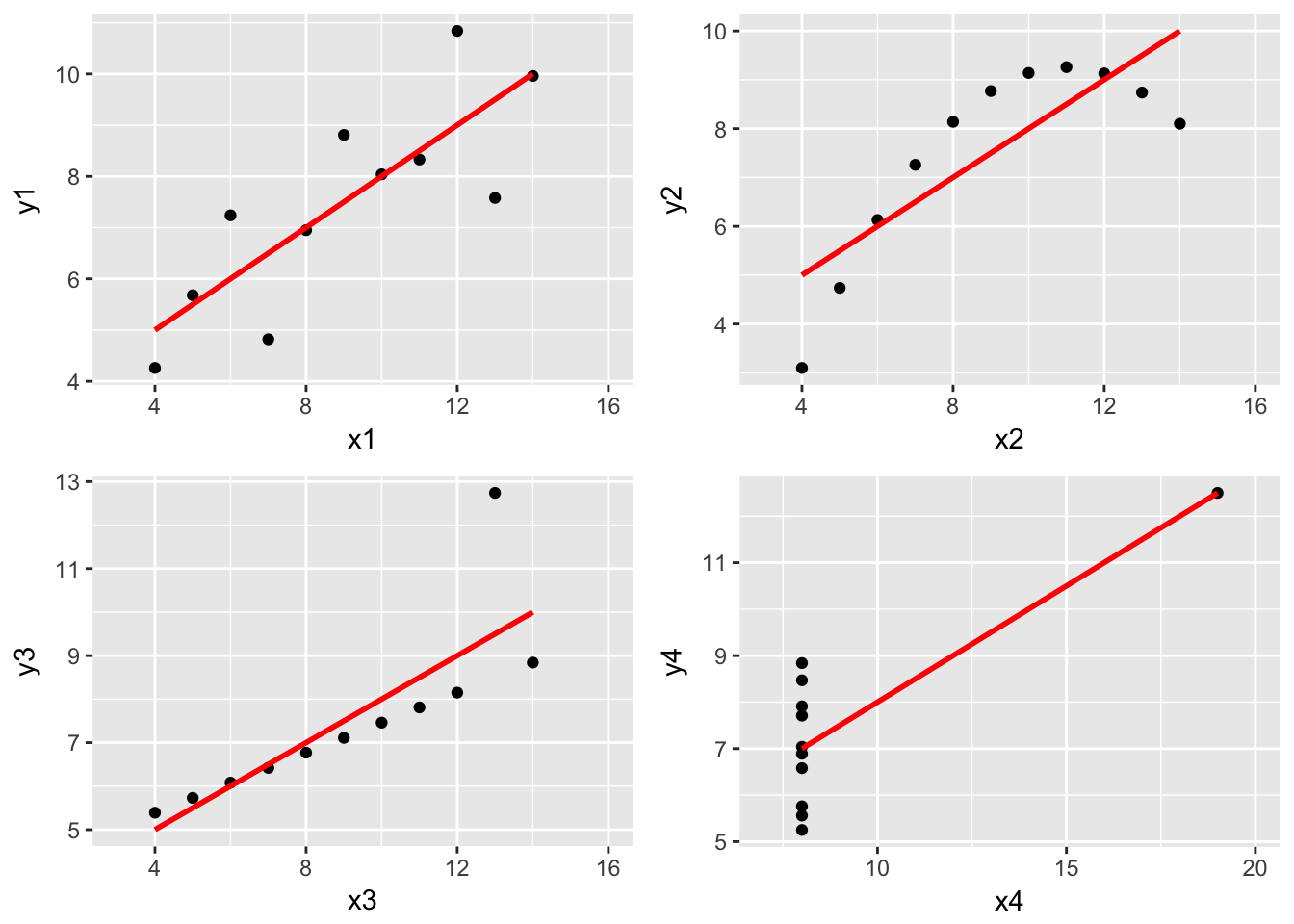# Multiple graphs with ggplot

So, I am learning how to use ggplot. Here is one my first attempts and at the same time I discover that you need to use the gridExtra package to layout multiople ggplots. I am using one of my favourite datasets: the Anscombe quartet

library(ggplot2)
library(grid)
library(gridExtra)

data(anscombe)

The quartet comprises four datasets that have nearly identical simple descriptive statistics, yet appear very different when graphed.

p1<-ggplot(anscombe,aes(x1,y1))+geom_point()+geom_smooth(method="lm",se=FALSE,color="red")+xlim(3,16)
p2<-ggplot(anscombe,aes(x2,y2))+geom_point()+geom_smooth(method="lm",se=FALSE,color="red")+xlim(3,16)
p3<-ggplot(anscombe,aes(x3,y3))+geom_point()+geom_smooth(method="lm",se=FALSE,color="red")+xlim(3,16)
p4<-ggplot(anscombe,aes(x4,y4))+geom_point()+geom_smooth(method="lm",se=FALSE,color="red")+xlim(7,20)

grid.arrange(p1,p2,p3,p4,ncol=2)We can see that the fitted lines are actually almost identical since the summary statistics are almost identical as well.

coefficients(lm(y1~x1,anscombe))
## (Intercept)          x1
##   3.0000909   0.5000909
coefficients(lm(y2~x2,anscombe))
## (Intercept)          x2
##    3.000909    0.500000
coefficients(lm(y3~x3,anscombe))
## (Intercept)          x3
##   3.0024545   0.4997273
coefficients(lm(y4~x4,anscombe))
## (Intercept)          x4
##   3.0017273   0.4999091